# Solving Oblique Triangles Using the Law of Cosines

Lesson Transcript
Instructor: Yuanxin (Amy) Yang Alcocer

Amy has a master's degree in secondary education and has taught math at a public charter high school.

After watching this video lesson, you will be able to use the law of cosines to help you find a missing side or missing angle of any kind of triangle. Also, you will see what oblique triangles are.

## Law of Cosines

This video lesson will show you how you can use the law of cosines, a formula to help you solve all kinds of triangles, to help you find the missing side or angle of any kind of triangle. The formula looks very similar to the Pythagorean Theorem, a^2 + b^2 = c^2, with just one difference. Here it is: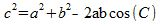The only difference between the law of cosines and the Pythagorean Theorem is that we have a minus 2ab cos (C). An easy way to remember this part is to remember a, b, and c again. We have a, b, and c again, so we have a 2 in front. See if you can think of other memory tricks to help you remember this formula.

Just like in the Pythagorean Theorem, our small letters a, b, and c stand for the sides of the triangle. But since this formula works for any kind of triangle, our letter c can be for any side of the triangle, not just the hypotenuse of a right triangle. The large letter C at the end stands for the angle C that is opposite side c.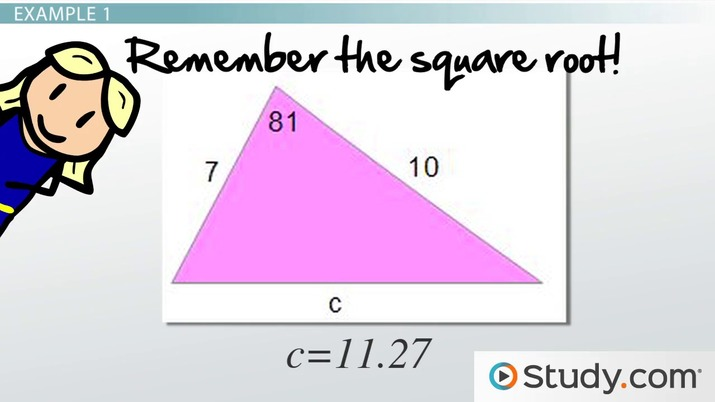An error occurred trying to load this video.

Try refreshing the page, or contact customer support.

Coming up next: Solving Real World Problems Using the Law of Cosines

### You're on a roll. Keep up the good work!

Replay
Your next lesson will play in 10 seconds
• 0:01 Law of Cosines
• 1:09 Oblique Triangles
• 1:59 Example 1
• 3:24 Example 2
• 5:36 Lesson Summary
Save Save

Want to watch this again later?

Timeline
Autoplay
Autoplay
Speed Speed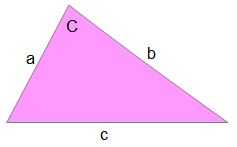## Oblique Triangles

In this video lesson, we are specifically looking at oblique triangles. These are triangles that are not right triangles. What kinds of triangles does this cover? It covers all kinds of triangles. It covers acute triangles, scalene triangles, obtuse triangles, and even equilateral triangles. Any kind of triangle that is not a right triangle is an oblique triangle.

Looking at the formula, we can see that it can help us to find the measurement of side c if we know the measurement of the other two sides, a and b, along with the angle opposite side c. We can also find the measure of angle C if we know the measurements of all three sides. We can solve for the measure of angle C by doing some algebraic rearranging of the formula.

Do you want to see a couple of examples of how this is done?

## Example 1

Let's look at this example, where we want to find the measurement of a missing side.

Find side c.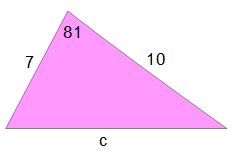If this triangle wasn't labeled in any way, we could simply label the side we want as side c since that will make our formula easier to use. Because the formula works for any triangle, it doesn't matter which side we label with a, b, or c. We can label it any way that will make our problem solving easier.

In this case, the side we want to find is already labeled as side c, which helps us out a lot. We can go ahead and label the other two sides as a and b. Again, it doesn't matter which is which. So, I will go ahead and label the 7 as side a and the 10 as side b. Our angle C, the angle opposite the side we want to find, is 81. Now, we have a = 7, b = 10, and C = 81. We plug in these values into our formula.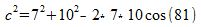Now we can evaluate the formula and then solve it. We get c^2 = 49 + 100 - 140 cos (81) = 149 - 21.9 = 127.1. Taking the square root, we get c = 11.27. So, our side measures about 11.27. When you are finding a missing side, don't forget to finish off by taking the square root to get side c by itself.

## Example 2

Now, let's look at an example where we find a missing angle. Remember what I said about how we can label our triangle so that it helps us to use the formula? Whatever angle we are looking for, we can label it as angle C, the side opposite it as side c, and the other two sides as side a and side b.

Find the measure of angle x.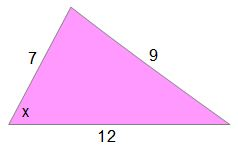To unlock this lesson you must be a Study.com Member.

### Register to view this lesson

Are you a student or a teacher?